## NCERT solutions for class 10 maths : Exercise Solutions

### Chapter 01-Real Numbers-Exercise Solutions

NCERT Book: Page Number 7- Exercise 1.1

Q.1. Use Euclid’s division algorithm to find the HCF of:
Since 225 > 135, we apply the division lemma to 225 and 135 to obtain
225 = 135 × 1 + 90
Since remainder 90 ≠ 0, we apply the division lemma to 135 and 90 to obtain
135 = 90 × 1 + 45
We consider the new divisor 90 and new remainder 45, and apply the division lemma to obtain
90 = 2 × 45 + 0
Since the remainder is zero, the process stops.
Since the divisor at this stage is 45,
Therefore, the HCF of 135 and 225 is 45.
(ii)196 and 38220
Since 38220 > 196, we apply the division lemma to 38220 and 196 to obtain
38220 = 196 × 195 + 0
Since the remainder is zero, the process stops.
Since the divisor at this stage is 196,
Therefore, HCF of 196 and 38220 is 196.
(iii)867 and 255
Since 867 > 255, we apply the division lemma to 867 and 255 to obtain
867 = 255 × 3 + 102
Since remainder 102 ≠ 0, we apply the division lemma to 255 and 102 to obtain
255 = 102 × 2 + 51
We consider the new divisor 102 and new remainder 51, and apply the division lemma to obtain
102 = 51 × 2 + 0
Since the remainder is zero, the process stops.
Since the divisor at this stage is 51,
Therefore, HCF of 867 and 255 is 51.
Q.2. Show that any positive odd integer is of the form 6q +1, or 6q + 3, or 6q + 5 , where q is some integer.
Answer: Let a be any positive integer and b = 6. Then, by Euclid’s algorithm,
a = 6q + r for some integer q ≥ 0, and r = 0, 1, 2, 3, 4, 5 because 0 ≤ r < 6.
Therefore, a = 6q or 6q + 1 or 6q + 2 or 6q + 3 or 6q + 4 or 6q + 5
Also, 6q + 1 = 2 × 3q + 1 = 2$k_{1}$ + 1, where $k_{1}$ is a positive integer
6q + 3 = (6q + 2) + 1 = 2 (3q + 1) + 1 = 2k2 + 1, where $k_{2}$ is an integer
6q + 5 = (6q + 4) + 1 = 2 (3q + 2) + 1 = 2k3 + 1, where $k_{3}$ is an integer
Clearly, 6q + 1, 6q + 3, 6q + 5 are of the form 2k + 1, where k is an integer.
Therefore, 6q + 1, 6q + 3, 6q + 5 are not exactly divisible by 2. Hence, these expressions of numbers are odd numbers.
And therefore, any odd integer can be expressed in the form 6q + 1, or 6q + 3,
or 6q + 5

Q.3. An army contingent of 616 members is to march behind an army band of 32 members in a parade. The two groups are to march in the same number of columns. What is the maximum number of columns in which they can march?

Answer: HCF (616, 32) will give the maximum number of columns in which they can march.
We can use Euclid’s algorithm to find the HCF.
616 = 32 × 19 + 8
32 = 8 × 4 + 0
The HCF (616, 32) is 8.
Therefore, they can march in 8 columns each.

Q.4. Use Euclid’s division lemma to show that the square of any positive integer is either of form 3m or 3m + 1 for some integer m.
[Hint: Let x be any positive integer then it is of the form 3q, 3q + 1 or 3q + 2. Now square each of these and show that they can be rewritten in the form 3m or 3m + 1.]
Answer: Let a be any positive integer and b = 3.
Then a = 3q + r for some integer q ≥ 0
And r = 0, 1, 2 because 0 ≤ r < 3
Therefore, a = 3q or 3q + 1 or 3q + 2
Or,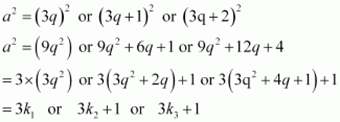Where $k_{1}$, $k_{2}$, and $k_{3}$ are some positive integers
Hence, it can be said that the square of any positive integer is either of the form 3m or 3m + 1.
Q.5. Use Euclid’s division lemma to show that the cube of any positive integer is of the form 9m, 9m + 1 or 9m + 8.
Answer: Let a be any positive integer and b = 3
a = 3q + r, where q ≥ 0 and 0 ≤ r < 3
$\therefore$ a = 3q or 3q +1 or 3q + 2
Therefore, every number can be represented as these three forms. There are three cases.
Case 1: When a = 3q,
$a^{3} =(3q)^{3} = 27q^{3} = 9(3q^{3})= 9m$
Where m is an integer such that m = $3q^{3}$
Case 2: When a = 3q + 1,
$a^{3} = (3q +1)^{3}$
$a^{3} = 27q^{3} + 27q^{2} + 9q + 1$
$a^{3} = 9(3q^{3} + 3q^{2} + q) + 1$
$a^{3} = 9m + 1$
Where m is an integer such that m = $(3q^{3} + 3q^{2} + q)$
Case 3: When a = 3q + 2,
$a^{3} = (3q +2)^{3}$
$a^{3} = 27q^{3} + 54q^{2} + 36q + 8$
$a^{3} = 9(3q^{3} + 6q_{2} + 4q) + 8$
$a^{3} = 9m + 8$
Where m is an integer such that $m = (3q^{3} + 6q^{2} + 4q)$
Therefore, the cube of any positive integer is of the form 9m, 9m + 1, or 9m + 8.
NCERT Book: Page Number 11- Exercise 1.2
Q.1. Express each number as product of its prime factors:
(i) 140 (ii) 156 (iii) 3825 (iv) 5005 (v) 7429
(i) 140 = 2 × 2 × 5 × 7 = $2^{2}$ × 5 × 7
(ii) 156 = 2 × 2 × 3 × 13 = $2^{2}$ × 3 × 13
(iii) 3825 = 3 × 3 × 5 × 5 × 17 = $3^{2} × 5^{2} × 17$
(iv) 5005 = 5 × 7 × 11 × 13
(v) 7429 = 17× 19 × 23
Q.2. Find the LCM and HCF of the following pairs of integers and verify that LCM × HCF = product of the two numbers.
(i) 26 and 91
(ii) 510 and 92
(iii) 336 and 54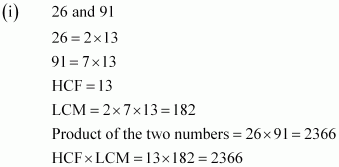Hence, product of two numbers = HCF × LCM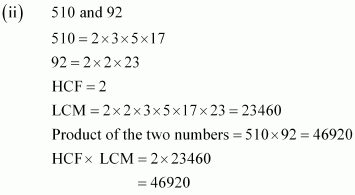Hence, product of two numbers = HCF × LCM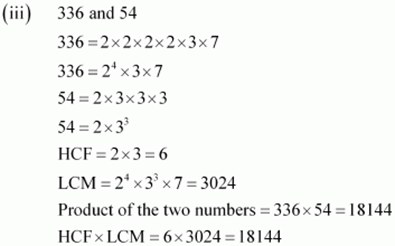Hence, product of two numbers = HCF × LCM
Q.3. Find the LCM and HCF of the following integers by applying the prime factorisation method.
(i) 12, 15 and 21
(ii) 17, 23 and 29
(iii) 8, 9 and 25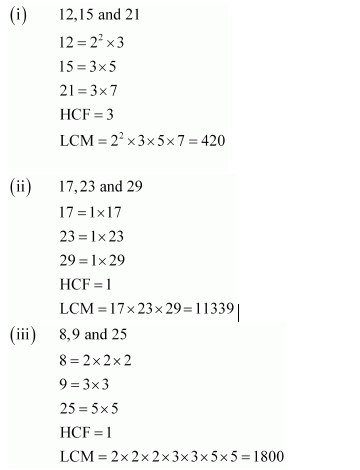Q.4. Given that HCF (306, 657) = 9, find LCM (306, 657).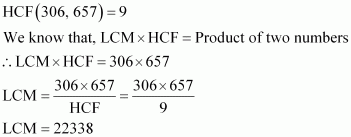Q.5. Check whether $6^{n}$ can end with the digit 0 for any natural number n.
Answer: If any number ends with the digit 0, it should be divisible by 10 or in other words, it will also be divisible by 2 and 5 as 10 = 2 × 5
Prime factorisation of 6n = (2 × 3)n
It can be observed that 5 is not in the prime factorisation of 6n.
Hence, for any value of n, 6n will not be divisible by 5.
Therefore, 6n cannot end with the digit 0 for any natural number n.

Q.6. Explain why 7 × 11 × 13 + 13 and 7 × 6 × 5 × 4 × 3 × 2 × 1 + 5 are composite numbers.
Answer: Numbers are of two types – prime and composite. Prime numbers can be divided by 1 and only itself, whereas composite numbers have factors other than 1 and itself.
It can be observed that
7 × 11 × 13 + 13 = 13 × (7 × 11 + 1) = 13 × (77 + 1)
= 13 × 78
= 13 ×13 × 6
The given expression has 6 and 13 as its factors. Therefore, it is a composite number.
7 × 6 × 5 × 4 × 3 × 2 × 1 + 5 = 5 ×(7 × 6 × 4 × 3 × 2 × 1 + 1)
= 5 × (1008 + 1)
= 5 ×1009
1009 cannot be factorised further. Therefore, the given expression has 5 and 1009 as its factors. Hence, it is a composite number.
Q.7. There is a circular path around a sports field. Sonia takes 18 minutes to drive one round of the field, while Ravi takes 12 minutes for the same. Suppose they both start at the same point and at the same time, and go in the same direction. After how many minutes will they meet again at the starting point?
Answer: It can be observed that Ravi takes lesser time than Sonia for completing 1 round of the circular path. As they are going in the same direction, they will meet again at the same time when Ravi will have completed 1 round of that circular path with respect to Sonia. And the total time taken for completing this 1 round of circular path will be the LCM of time taken by Sonia and Ravi for completing 1 round of circular path respectively i.e., LCM of 18 minutes and 12 minutes.
18 = 2 × 3 × 3
And, 12 = 2 × 2 × 3
LCM of 12 and 18 = 2 × 2 × 3 × 3 = 36
Therefore, Ravi and Sonia will meet together at the starting pointafter 36 minutes.

NCERT Book Page Number: 14- Exercise 1.3
Q.1. Prove that $\sqrt{5}$ is irrational.
Answer: Let $\sqrt(5)$ is a rational number.
Therefore, we can find two integers a, b (b ≠ 0) such that $\sqrt{5}= \frac{a}{b}$
Let a and b have a common factor other than 1. Then we can divide them by the common factor, and assume that a and b are co-prime.
$a = \sqrt{5} b$
$a^{2} = 5 b^{2}$

Therefore, $a^{2}$ is divisible by 5 and it can be said that a is divisible by 5.
Let a = 5k, where k is an integer
$(5k)^{2} = 5 b^{2}$
This means that$b^{2}$ is divisible by 5 and hence, b is divisible by 5.
This implies that a and b have 5 as a common factor.
And this is a contradiction to the fact that a and b are co-prime.
Hence, cannot be expressed as or it can be said that is irrational.
Q.2. Prove that $3 + 2\sqrt5$ is irrational.

Answer: Let $3 + 2\sqrt5$ is rational.

Therefore, we can find two integers a, b (b ≠ 0) such that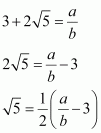Since a and b are integers, $\frac{1}{2}(\frac{a}{b}-3)$ will also be rational and therefore,is rational.
This contradicts the fact that $\sqrt(5)$ is irrational. Hence, our assumption that $3 + 2\sqrt5$ is rational is false. Therefore, $3 + 2\sqrt5$ is irrational.